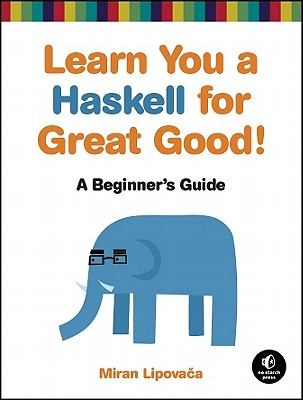I have spent the past 4-6 months playing with Haskell on the side. While I've yet come to the conclusion that one paradigm of programming is inherently better than the other, I have at least found it very intellectually rewarding and exciting to take a step back, and try to implement algorithms, data structures, and other hackings using a functional programmer's mindset. I can't say that I've fully grasped everything but I imagine it's just a function of time.

First, I highly recommend the following book: "Learn You a Haskell for Great Good"I chose a few random examples to demonstrate some of the cool examples that made me enjoy Haskell

Simple Functions

f x y = x*x + y*y 	-- simple function of x and y
g y = f 0 Y 		-- partial solved function

h :: Int -> Int -> Int -- typed function
h x y = x*x + y*y

i :: Num a => a -> a-> a -- any Numeric type
i x y = x*x + y*y

main = do
print (f 2 3)
print (g 3)
print (h 2 3)
-- (h 2.3 4.2) would fail
print (i 2 3.4)


Folding

main = do
print (foldl (+) 0 [1, 2, 3]) -- summation using foldl


Use of Gaurds to create Piecewise Functions:

guess x
| x > 27 = "Too high!"
| x < 27 = "Too low!"
| otherwise = "Correct!"

main = do
print (guess 100)
print (guess 0)
print (guess 27)


Filters:

main = do
print (filter even [1..10])
print (filter (>5) [1..10])


List Comprehensions, ∀x∈S, p(x):

-- ∀x∈S, p(x)
-- [ x | x <- s, p x ]

p = [ x+5 | x <- [1,2,3] ]
f x y = x + y

main = do
print p
print ([ x+5 | x <- [1,2,3] ])
print ([ x | x <- [2..10], 10 mod x == 0])
print ([ team ++ " " ++ player |
team <- ["red", "blue"],
player <- ["soldier", "pyro", "scout"] ])

print ([ x | x<-[1..10], even x ])

print ([ f x y
| x <- [1..10]
, y <- [1..10]])


Function Composition:

main = do
-- f(g(h(k(x)))) - ugly
-- (f.g.h.k)(x) - pretty
print ((not.odd) 4)

let f x = 2 * x + 3
let g x = x * x
let h x = sin x

print ((f.g.h) 3)
print (f (g (h 3)))


Function Examples using Let and Where:

slope (x1,y1) (x2,y2) = let dy = y2-y1
dx = x2-x1
in dy/dx

slope2 (x1,y1) (x2,y2) = dy/dx
where dy = y2-y1
dx = x2-x1

slope3 (x1,y1) (x2,y2) = (y2 - y1) / (x2 - x1)

main = do
print (slope (1,1) (2,2))
print (slope2 (1,1) (2,2))
print (slope (1,3) (10,4))
print (slope (-1,1) (0, 0))


Fibonacci Sequence Implementations:

-- simple, naive implementation
slow_fib :: Int -> Integer
slow_fib 0 = 1
slow_fib 1 = 1
slow_fib n = slow_fib (n-2) + slow_fib (n-1)

-- a memoized version. The magic lies in the "map fib [0 ..]" which is
-- the fibonacci sequence mapped to an infinite list! ^_^ which is only
-- possible due to the lazy nature of Haskell.
memoized_fib :: Int -> Integer
memoized_fib = (map fib [0 ..] !!)
where fib 0 = 1
fib 1 = 1
fib n = memoized_fib (n-2) + memoized_fib (n-1)


Binary Tree:

This was my first attempt at implementing. I have since found more eloquent solutions :)

data Tree = Node { value::Int, left::Tree, right::Tree } | Null deriving (Show, Eq)

total :: Tree -> Int
total Null = 0
total node = value node + total (left node) + total(right node)

size :: Tree -> Int
size Null = 0
size node = 1 + size (left node) + size (right node)

add :: Int -> Tree -> Tree
add v Null = (Node v Null Null)
| v > (value node) = Node (value node) (left node) (add v (right node)) -- insert into right subtree
| v < (value node) = Node (value node) (add v (left node)) (right node) -- insert into left subtree
| otherwise = node -- value is(value node)new so add it


Fizz Buzz:

fizzbuzz x
| (mod x 15) == 0 = "FizzBuzz"
| (mod x 3) == 0  = "Fizz"
| (mod x 5) == 0  = "Buzz"
| otherwise = show x

main = print(map fizzbuzz [1..100])


PI Gregory Series:

piSum :: Int -> Double
piSum n = sum (map f [1..n])

f :: Int -> Double
f x = 4 * (-1)^(x+1) / (2*k - 1)
where k = fromIntegral x

main = do
print "pi sum 1"
print (piSum 1)
print (piSum 2)
print (piSum 5)
print (piSum 10)
print (piSum 100)
print (piSum 1000)
print (piSum 10000)
print (piSum 100000)


Quick Sort:

qsort [] = []

qsort (p:xs) = (qsort lesser) ++ [p] ++ (qsort greater)
where
lesser  = filter (< p) xs
greater = filter (>= p) xs

main = print (qsort [20,3,14,6,1,10])


Triangles:

triangles n = [(a,b,c)
| c<-[1..n]
, b<-[1..c]
, a<-[1..b]
, a^2 + b^2 == c^2]

main = do
print (triangles 10)
print (triangles 15)
print (triangles 100)


Drop piece into Connect 4 Column:

numEmpty :: [Int] -> Int
numEmpty board = length $filter (\x -> x == 0) board addToColumn :: Int -> [Int] -> [Int] addToColumn val board = xs ++ [val] ++ ys where n = (numEmpty board) xs = replicate (n - 1) 0 ys = snd (splitAt n board) -- simulate dropping a piece into a connect 4 board column main = do let board = [0,0,0,0,0,1,-1,1] print board print$ addToColumn 1 board
-- outputs [0,0,0,0,1,1,-1,1]


Utf8:

 -- 値をゼロにする (utf8 comment works
f x = 0

e = exp 1
シグモイド :: Double -> Double
シグモイド x = 1 / (1 + e**(-x))

main = do
print (f 10)
print (シグモイド 0)